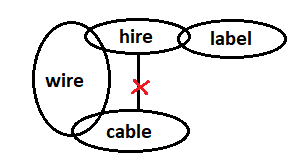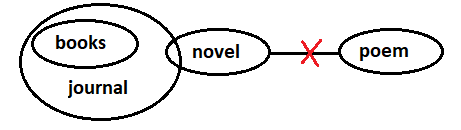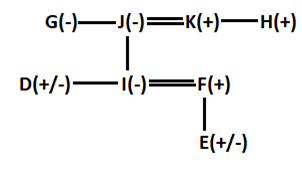# SBI Clerk Prelims Reasoning Ability Questions 2021 (Day-51)

Dear Aspirants, Our IBPS Guide team is providing new series of Reasoning Questions for SBI Clerk Prelims 2021 so the aspirants can practice it on a daily basis. These questions are framed by our skilled experts after understanding your needs thoroughly. Aspirants can practice these new series questions daily to familiarize with the exact exam pattern and make your preparation effective.

Start Quiz

Syllogism

Direction (1-3): In each question below are given some statements followed by some conclusions. You have to take the given statements to be true even if they seem to be at variance with commonly known facts. Read all the conclusions and then decide which of the given conclusions logically follows/follow from the given statements, disregarding commonly known facts.

1) Statements
:

Few cable are wires.

Few hire are wires.

No cable is hire

Some label are hire.

Conclusions:

I). Some label are not cable.

II). Some label are cable.

A.Only conclusion I is true

B.Only conclusion II is true

C.Either conclusion I or conclusion II is true

D.Neither conclusion I nor conclusion II is true

E.Both conclusion I and II are true

2) Statements
:

Mostly catch are batch.

Some watch are batch.

Every catch are match.

Conclusions:

I). All watch can never be match

II). Some match being batch is a possibility

A.Only conclusion I is true

B.Only conclusion II is true

C.Either conclusion I or conclusion II is true

D.Neither conclusion I nor conclusion II is true

E.Both conclusion I and II are true

3) Statements:

All books are journal

Few Journal are novel

All novels are poem

Conclusions:

I). Some poem are journal

II). Some poem are not books is a possibility

A.Only conclusion I is true

B.Only conclusion II is true

C.Either conclusion I or conclusion II is true

D.Neither conclusion I nor conclusion II is true

E.Both conclusion I and II are true

Blood relation

Direction (4-6): Study the following information carefully and answer the questions given below:

There are 8 members D, E, F, G, H, I, J and K in a family which consists of only two married couples. There is no single parent in the family. I is married daughter of J, who has two children. J is the sister-in-law of H. E is the only child of F. G is the sister-in-law of grandfather of E. D is younger than K, who doesn’t have sister.

4) How is E related to the father of D?

A.Granddaughter

B.Daughter

C.Son

D.Nephew

E.Can’t be determined

5) If F doesn’t have brother in law, how is D related to sister in law of H?

A.Sister

B.Daughter

C.Mother

D.Sister in law

E.Can’t be determined

6) How many male members are there in the family?

A.Two

B.Three

C.Four

D.Can’t be determined

E.None of these

Inequality

Direction (7-9): In these questions, the relationship between different elements is shown in the statement. The statements are followed by two conclusions. Choose the correct Answer given below:

7) Statements:

Z ≤ E < M = T ≥ H; Y > S > W ≤ H

Conclusions:

I). T ≥ Y

II). M < Y

A.Only conclusion I is true

B.Only conclusion II is true

C.Either conclusion I or conclusion II is true

D.Neither conclusion I nor conclusion II is true

E.Both conclusion I and II are true

8) Statements:

A > S ≥ D = G; K < F ≤ T < G; W ≥ R > K

Conclusions:

I). S > K

II). D < W

A.Only conclusion I is true

B.Only conclusion II is true

C.Either conclusion I or conclusion II is true

D.Neither conclusion I nor conclusion II is true

E.Both conclusion I and II are true

9) Statements:

X ≥ P > Q > D; L ≤ V = M; D < R ≤ L

Conclusions:

I). D < X

II). M ≥ R

A.Only conclusion I is true

B.Only conclusion II is true

C.Either conclusion I or conclusion II is true

D.Neither conclusion I nor conclusion II is true

E.Both conclusion I and II are true

10) How many such pairs of the letter are there in the word ‘ADHESIVE’ each of which has as many letters between them in the word as they have between them in the English alphabetical series (both in the backward and forward direction)?

A.One

B.Two

C.Three

D.Four

E.NoneDirections (4-6):